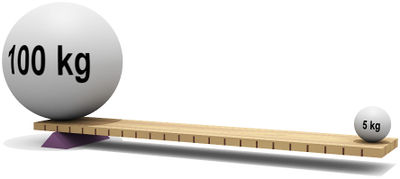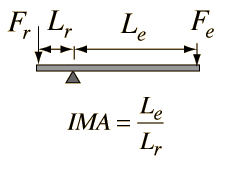# LeverFigure 1: An example of how a flat board can act like a lever. The fulcrum is triangular piece under the board that the whole system rotates around. The force from gravity on the 100 kg weight is much higher than the 5 kg weight, but the length at which the force is acting from the pivot (fulcrum) balances these forces out in terms of torque.

A lever is a type of simple machine, which creates a mechanical advantage to perform tasks by changing the magnitude and/or direction of forces. A lever needs a fulcrum (see figure 1), which is the central pivot for the system to manipulate forces. A lever also needs some sort of rigid bar or rod placed in some position on the fulcrum to be functional.

While levers may seem magical in that they change how much force is needed to do a job—they don't change the work or energy needed. Like all simple machines, people use the physics of levers to their advantage, but there's a trade off for using less force—the force must be applied over a greater distance.

Many everyday tools use levers and fulcrums in their use, such as nail clippers, pliers, scissors, wheelbarrows, fishing rods, crow bars, and nutcrackers, just to name a few. The principles behind levers can also be explored by seeing how hard of a push is needed to open a door at different distances from its hinges.Figure 2: The mechanical advantage of a lever. The subscript r represents the resistance force caused by a load, and the subscript e represents the effort, or input force. 

Levers use the principle of torque (the measure of the force applied on something at a distance from a pivot), in their operation. With levers, the position of the fulcrum determines the distribution of the balanced torques on either side, as can be seen in Figure 1 (or in the PhET at the bottom of the page).

From conservation of energy principles, the output energy of a system can not be larger energy inputted. The balanced equation looks like , which can be rearranged to define the mechanical advantage. For a lever, the ideal mechanical advantage is the ratio of the long side to the short side, as shown in Figure 2. Some levers are force multiplying (motion through a greater distance creates more force, like a crow bar) and some levers are distance multiplying (greater force through a shorter distance multiplies the force, like a fishing rod).

## Types of Levers

There are 3 types (or classes) of levers:

• Class-1 levers have the fulcrum in the middle, and the applied force is up or down on one of the ends, with the output force being in the opposite direction on the other end. Examples of class-1 levers include seesaws and scissors. These can be either force multiplying or distance multiplying.
• Class-2 levers have the fulcrum at one of the ends of the lever, and the applied force is at the other end. The resistance is located in the middle, and travels in the same direction as the applied force. An example of a class-2 lever is a wheelbarrow. These levers are always force multiplying.
• Class-3 levers are much like class-2 levers, but the applied force is in the middle rather than at the end. This means that the force a person needs to apply is larger than the output force (the mechanical advantage is less than 1), but this gives increased control of the tool. Examples of class-3 levers include tweezers, tongs, and fishing rods. These levers are always distance multiplying.

## Phet Simulation

The University of Colorado has graciously allowed us to use the following Phet simulation. Explore this simulation to see how forces acting on different parts of a beam create torques that can balance out. This is a class 1 lever.